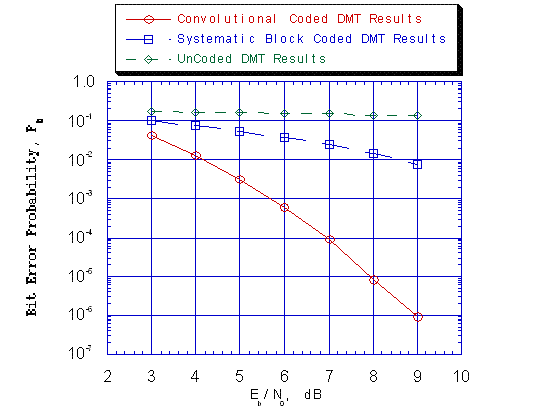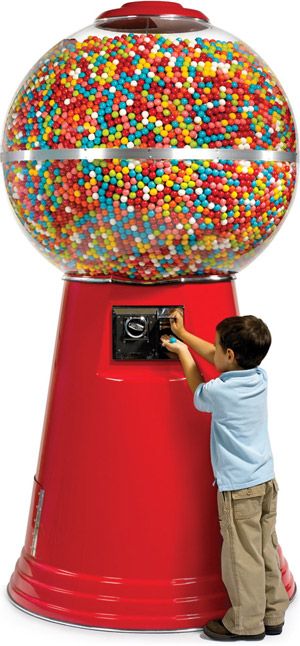&#215;

# Accuracy and Precision

Author: Peter Anderson

## Accuracy and precision

Accuracy is how close a measurement comes to the truth, represented as a bullseye above.  Accuracy is determined by how close a measurement comes to an existing value that has been measured by many, many scientists and recorded in the CRC Handbook.

Precision is how close a measurement comes to another measurement.  Precision is determined by a statistical method called a standard deviation.  Standard deviation is how much, on average, measurements differ from each other.  High standard deviations indicate low precision, low standard deviations indicate high precision.This classic diagram illustrates what combinations of accuracy and precision exist.  The precise measurements both exhibit tight grouping near some portion of the dartboard.  The accurate measurements are near the center.

To determine if a value is accurate compare it to the accepted value.  As these values can be anything a concept called percent error has been developed.  Find the difference (subtract) between the accepted value and the experimental value, then divide by the accepted value.

% error = (accepted - experimental) / accepted *100%

To determine if a value is precise find the average of your data, then subtract each measurement from it.  This gives you a table of deviations.  Then average the deviations.  This will give you a value called uncertainty.  A plus or minus value that says how precise a measurement is.

deviation = (average - actual)

standard deviation = (deviations for all measurements added together) / number of measurements

## Error, systematic, random, and gross

Error refers to a lack of accuracy, precision, or both.  Systematic and gross error are controllable, random error is not.  Knowing the type of error can lead to a solution.

Systematic error arises from the experimental design and affects the result in one direction, up or down.

Gross error arises from an undetected mistake that causes the measurement to be greatly different than the average.  This measurement is called an outlier.  If it is detected it is called a mistake or accident and the experiment is repeated.

Random error arises from nature and affects the result in two directions up and down.

Let's look at what these look like in your data sets.

Random ErrorThese results show the scattering of the data above and below the line.  Since the data is "all over the place" (low precision) or above and below the line it is classified as random.  Scientists have no way to fix random error, so we tell it like it is and report it with standard deviations and R2 values, which come from standard deviations.  Precision is affected, but accuracy is preserved.

Systematic errorThis graph shows systematic error in the blue line.  It is consistently above the red line, indicating that something is wrong.  When an experiment generates a result that is greatly above or below a measurement (low accuracy, high precision) an examination for systematic error is called for.  Accuracy is damaged, precision is not.

Gross errorSometimes in science you mess up.  It happens.  Most of the time scientists notice, shrug their shoulders and repeat the experiment.  Sometimes they don't notice, this is called a gross error.  It looks like this graph if there's only one.  Nice regular, somewhat linear data and then that one point that you wish would go away.

If you did not notice anything go wrong it would be dishonest not to record the outlier.  It is statistically difficult to declare a point an outlier.  It must be 3 standard deviations away from what it should be and that is a high bar.  Sometimes scientists deal with these by repeating that portion of an experiment and replace the data.  Sometimes they just get rid of it.  These damage accuracy and precision.

So there's three types of error that can happen.

Systematic - all a little up or a little down

Random - all a little up and a little down

Gross - one part very much up or very much down.

## Systematic and random error

A short, clear description

## Counting the gumballsLet's say you are trying to count the gumballs in this giant gumball machine.  If you assume that the dome is a sphere and calculate its volume and then the volume of an individual gumball you can come up with a value that will always be higher than the actual number of gumballs.  This experiment makes assumptions about gumballs in the machine that are incorrect.  There is space between the gumballs that must be accounted for, space at the top, the glass container has a thickness, and the experiment neglects the delivery chute that contains some gumballs.  These are systematic errors arising from assumptions.  This experiment will give quite precise values time after time as gumballs are very close to the same size and the size of the dome doesn't change much, but the accuracy will never be there until the whole experiment is redesigned.

So, lets redesign it.  If the gumballs are poured out into buckets of equal size and then the buckets counted it should give the same answer.  After doing this three times the results of 12.25 buckets, 11.75 buckets, and 11.25 buckets are received.  This error is random error owing to how well the gumballs settle in the buckets.  It affects precision, but the spread of the data when averaged can give an accurate result.

What if someone stole a bucket?  What if the count is off?  What if the first few buckets are full to the brim, but the rest aren't?  What if some gumballs spill out and go rolling accross the street and into the gutter, forever lost?  What if there are jellybeans in the middle of the gumball machine?

and you don't notice.

These scenarios all cause gross error which would appear as an outlier on the graph.  It damages accuracy and precision if you leave the point in, but there would definitely be something wrong if there were suddenly 6 buckets after the other results were achieved.